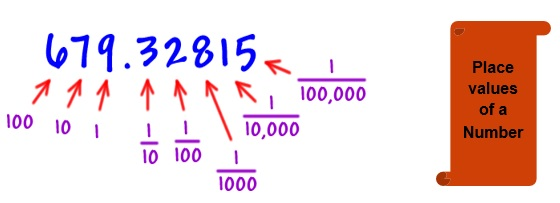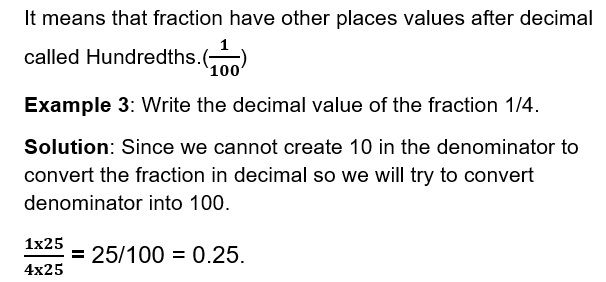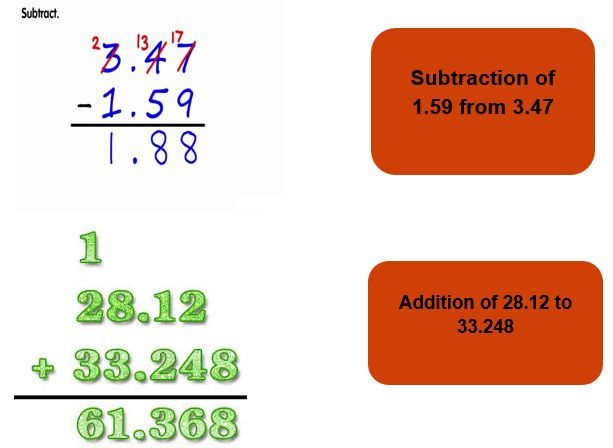What are Decimals? We all come across a lot of numbers which are not integers. So what are they and how can be they represented and what role does this play in mathematics? We will learn about these questions and try to find the relevant answers

A fraction whose denominator is a power of ten and whose numerator is expressed by figures placed to the right of a decimal point is decimal.

In simple words we all know that on dividing the fractions, we get remainder which means that the number cannot be further divided but it’s not true. On further division of number we get decimal values.

• Decimals also have place values like Tenths (1/10), Hundredths (1/100), etc.Example1: Write the number in decimal form

1. a) 5+10+2/10 b) 4+5+7/10

Solution: a) 2/10 can be written as 0.2

Hence the expression becomes 5+10+0.2 = 15.2

1. b) 7/10 becomes 0.7

Hence the expression becomes 4+5+0.7 = 9.7

• As we know that fractions can be changed to decimals, it is also 100 percent true that decimals can also be changed to their fraction values.

Example 2: Convert the following to its decimal or fraction value:

1. a) 1/2 b) 4.5

Solution: a) 1/2 can be changed to 5/10 by writing its equivalent fraction and hence we can write it as 0.5

1. b) 4.5 can be written as 4+5/10 which will be 40/10+5/10 which will be 45/10 and after reducing we get 9/2 as the fraction.

Hundredths

We all know that 1 m = 100 cm.

And also we can say that 1 cm =1/100 m

Here 1/100 represents a fraction of a meter and hence it will surely have a decimal value which is actually 0.01.Comparing Decimals

Comparison of decimal values involves few steps which are:

Step 1: Check the whole number.

Step 2: Check the Tenths place

Step 3: Check the Hundredths place

Example 4: Compare the following:

1. a) 45.25 and 14.23 b) 32.52 and 32.56

Solution: a) Here on comparing the whole number 45 and 14 we get that 45.25 > 14.23

1. b) Here both the whole numbers are same therefore we check the tenth place which is 5 in both the cases and hence on checking the hundredth place we get 2 and 6 which means 32.56 > 35.5

Using Decimals

Money:

1 Rupee = 100 Paisa which means

1 Paisa = 1/100 Rupee

Length:

1 m =100 cm

1 cm = 1/100 m

Weight:

1 kg = 1000g

1 g = 1/1000 kg.

Example 5: Rani had Rs. 18.50. She bought one ice-cream for Rs 11.75. How much money does she have now?

Solution: Money with Rani initially = Rs.18.50

Cost of Ice cream = 11.75

Money left = Rs. 18.50 – Rs. 11.75 = Rs. 5.25

The addition and subtraction operations on decimals are exactly same as that of integers. Let’s learn from an example.Practice Questions

Q1) Rahgav bought vegetables weighing 9 kg. Out of this, 3 kg 500 g is onions, 2 kg 200 g is tomatoes and the rest is Green vegetables. What is the weight of the green vegetables?

1. a) Rs. 20.45 to Rs. 78.65
2. b) 1m 20 cm to 600cm
3. c) 2kg 30 g to 4kg 200 g

Q3) Nasreen bought 3 m 20 cm cloth for her shirt and 2 m 5 cm cloth for her trouser. Find the total length of cloth bought by her.

Q4) Express in Rupee as decimals

1. a) 45 paisa b) 12 paisa c) 100 paisa d) 30 paisa

Q5) Which is greater?

1. 98 and 1.098
2. 2 or 4.3
3. 23 or 4.22
4. 1 or 9.01

Q6) Write the following in decimal words:

1. 45
2. 62
3. 24
4. 369

Recap

• Every fraction with denominator 10 can be written in decimal notation and vice-versa.
• Every fraction with denominator 100 can be written in decimal notation and vice-versa.

• In the place value table, as we go from left to the right, the multiplying factor becomes 1/10 of the previous factor.
• Every decimal can be written as a fraction.
• Any two decimal numbers can be compared among themselves. The comparison can start with the whole part. If the whole parts are equal then the tenth parts can be compared and so on.
• The place value 1/10 signifies the decimal value 0.1 whereas the place value 1/100 signifies the decimal value 0.01.